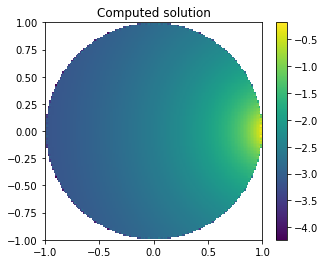# Solving a Laplace problem with Dirichlet boundary conditions¶

### Background¶

In this tutorial, we will solve a simple Laplace problem inside the unit sphere with Dirichlet boundary conditions. Let $\Omega$ be the unit sphere with boundary $\Gamma$. Let $\nu$ be the outward pointing normal on $\Gamma$. The PDE and boundary conditions are given by

\begin{align} \Delta u &= 0&&\text{in }\Omega,\\ u &= f&&\text{on }\Gamma. \end{align}

The boundary data is a source $\hat{u}$ located at the point $(0.9,0,0)$. $$\hat{u}(\mathbf x)=\frac{1}{4\pi\sqrt{(x-0.9)^2+y^2+z^2}}.$$

For this example, we will use a direct integral equation of the first kind. Let $$g(\mathbf x,\mathbf y) = \frac{1}{4\pi |\mathbf x-\mathbf y|}$$ be the Green's function for Laplace in three dimensions. From Green's representation theorem, it follows that every harmonic function $u$ in $\Omega$ satisfies

$$u(\mathbf x) = \int_{\Gamma} g(\mathbf x,\mathbf y)\frac{\partial u(\mathbf y)}{\partial \nu(\mathbf{y})}\mathrm{d}\mathbf y-\int_{\Gamma}\frac{\partial g(\mathbf x,\mathbf y)}{\partial \nu(\mathbf{y})}u(\mathbf y)\mathrm{d}\mathbf y,~\mathbf x\in\Omega\setminus\Gamma$$

or equivalantly

$$u(\mathbf x) = \left[\mathcal{V}\frac{\partial u(\mathbf y)}{\partial \nu(\mathbf{y})}\right] (\mathbf{x}) - \left[\mathcal{K}u\right] (\mathbf{x}),~\mathbf x\in\Omega\setminus\Gamma,$$

where $\mathcal{V}$ and $\mathcal{K}$ are the single and double layer potential operators.

Taking the limit $\mathbf x\rightarrow \Gamma$ we obtain the boundary integral equation

$$\left[\mathsf{V}\frac{\partial u}{\partial n}\right] (\mathbf x)=\left[(\tfrac12\mathsf{Id}+\mathsf{K})u\right] (\mathbf x),~\mathbf x\in\Gamma.$$

Here, $\mathsf{V}$ and $\mathsf{K}$ are the single and double layer boundary operators.

### Implementation¶

We now demonstrate how to solve this problem with Bempp. We begin by importing Bempp and NumPy.

In :
import bempp.api
import numpy as np


We next define a mesh or grid. For this problem, we will use the built-in function sphere that defines a simple spherical grid.

In :
grid = bempp.api.shapes.sphere(h=0.1)


We now define the spaces. For this example we will use two spaces: the space of continuous, piecewise linear functions; and the space of piecewise constant functions. The space of piecewise constant functions has the right smoothness for the unknown Neumann data. We will use continuous, piecewise linear functions to represent the known Dirichlet data.

In :
dp0_space = bempp.api.function_space(grid, "DP", 0)
p1_space = bempp.api.function_space(grid, "P", 1)


We can now define the operators. We need the identity, single layer, and double layer boundary operator.

In :
identity = bempp.api.operators.boundary.sparse.identity(
p1_space, p1_space, dp0_space)
dlp = bempp.api.operators.boundary.laplace.double_layer(
p1_space, p1_space, dp0_space)
slp = bempp.api.operators.boundary.laplace.single_layer(
dp0_space, p1_space, dp0_space)


We now define the GridFunction object on the sphere grid that represents the Dirichlet data.

In :
@bempp.api.real_callable
def dirichlet_data(x, n, domain_index, result):
result = 1./(4 * np.pi * ((x - .9)**2 + x**2 + x**2)**(0.5))

dirichlet_fun = bempp.api.GridFunction(p1_space, fun=dirichlet_data)


We next assemble the right-hand side of the boundary integral equation, given by $$(\tfrac12\mathsf{Id}+\mathsf{K})u.$$

In :
rhs = (.5 * identity + dlp) * dirichlet_fun


We now solve the linear system using a conjugate gradient (CG) method.

In :
neumann_fun, info = bempp.api.linalg.cg(slp, rhs, tol=1E-3)


We now want to provide a simple plot of the solution in the $(x,y)$ plane for $z=0$. We first define points at which to plot the solution.

In :
n_grid_points = 150
plot_grid = np.mgrid[-1:1:n_grid_points*1j, -1:1:n_grid_points*1j]
points = np.vstack((plot_grid.ravel(),
plot_grid.ravel(),
np.zeros(plot_grid.size)))


The variable points now contains in its columns the coordinates of the evaluation points. We can now use Green's representation theorem to evaluate the solution on these points. Note in particular the last line of the following code. It is a direct implementation of Green's representation theorem.

In :
slp_pot = bempp.api.operators.potential.laplace.single_layer(
dp0_space, points)
dlp_pot = bempp.api.operators.potential.laplace.double_layer(
p1_space, points)
u_evaluated = slp_pot * neumann_fun - dlp_pot * dirichlet_fun


We now plot the 2D slice of the solution. For a full three dimensional visualization, Bempp can export the data to Gmsh. Since the solution decays quickly, we use a logarithmic plot.

In :
# The next command ensures that plots are shown within the IPython notebook
%matplotlib inline

# Filter out solution values that are associated with points outside the unit circle.
u_evaluated = u_evaluated.reshape((n_grid_points,n_grid_points))

# Plot the image
import matplotlib
matplotlib.rcParams['figure.figsize'] = (5.0, 4.0)

from matplotlib import pylab as plt

plt.imshow(np.log(np.abs(u_evaluated.T)), extent=(-1,1,-1,1))
plt.title('Computed solution')
plt.colorbar()

Out:
<matplotlib.colorbar.Colorbar at 0x7f4835f5a310>